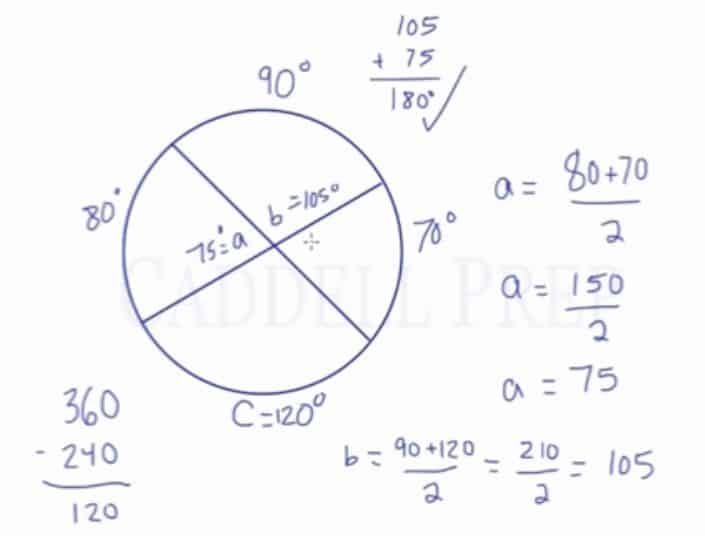In this video, we are going to look at arcs and angles formed by intersecting chords.

When two chords intersect, they form several angles and arcs. Vertical angles are congruent, but the arcs across from these angles are not. However, there is a relationship between the arcs and the angles. If we label the angle as$a$, and the arcs as$x$ and$y$, then the following is true:$a=\frac{x+y}{2}$

For example:
When given a circle with 4 arcs; 90, 80, 70, and$c$; and angles$a$ and$b$, we must solve for the missing variables. In this example,$a$ is the average of 80 and 70. So:$a=\frac{80+70}{2}=\frac{150}{2}=75$

To find the value of$c$, we know that the total sum of all the arcs is 360, so$360-240=120$.

Finally:$b=\frac{90+120}{2}=\frac{210}{2}=105$

You may have also noticed that the chord is a straight line, meaning that the two angles$a$ and$b$ would have to add up to 180.## Video-Lesson Transcript

In this lesson, we’re going to take a look at arcs and angles formed by intersecting chords.

In this circle, let’s draw two chords which intersect. When they intersect, they form a whole bunch of angles. There are two horizontal angles so they are equal. We have another two vertical angles which are also equal.

Here, we also have a number of arcs formed. We have two arcs facing each other horizontally and they are not equal. These two are not of the same length. The angles across from them are equal but the arcs do not equal each other.

Likewise, we have two arcs facing each other vertically. They also do not necessarily equal each other. The angles across from them are equal because they are vertical. But these two arcs are not equal to each other.

There is, however, relationships between these angles and the arcs.

For example, let’s take a look at the angles and arcs facing each other horizontally.

Let’s say the measure of one arc is$x^{\circ}$ and the measure of the other arc is$y^{\circ}$. The measure of the angles is$a^{\circ}$ since they are equal.

The measure of$a^{\circ}$ angle is not necessarily the same as$x^{\circ}$ arc nor$y^{\circ}$ arc.

However, what we know is that$a^{\circ}$ is the average of the two arcs.$a = \dfrac{x + y}{2}$

We could do the same with the angles and arcs facing each other vertically.

Let’s say the angle is$b^{\circ}$ and the two arcs are$w^{\circ}$ and$z^{\circ}$.

The angle$b^{\circ}$ is also the average of the two arcs.$b = \dfrac{w + z}{2}$

In this example we have the measure of three arcs:$80^{\circ}$,$90^{\circ}$,$70^{\circ}$, and a missing arc$c$. We also have to find out what these angles are$a$ and$b$.

When we look at this, we can say that angle$a$ is the same as the angle it faces. And these angles intercept$80^{\circ}$ arc and$70^{\circ}$ arc. So,$a$ is the average of these two arcs.$a = \dfrac{80 + 70}{2}$$a = \dfrac{150}{2}$$a = 75$

Next, we’ll find$c$.

We know that when we go all around the circle, we end up with$360^{\circ}$. So, all of these arcs should add up to$360^{\circ}$.

Now, we can solve for$c$$360 - (80 + 90 + 70)$$360 - 240$$c = 120$

Angle$b$ which represents two angles facing each other which intercepts$90^{\circ}$ arc and$120^{\circ}$ arc. So,$b$ is the average of these two.$b = \dfrac{90 + 120}{2}$$b = \dfrac{210}{2}$$b = 105$

You might notice that one chord is a straight line. So, the two angles should add up to$180^{\circ}$.

If we were to check it, we’ll do$105 + 75 = 180$

We can use this as a back up to check.

This is an example of a question we can have that involves intersecting chords, the measurements of their arcs, and also the measurements of the angles formed.